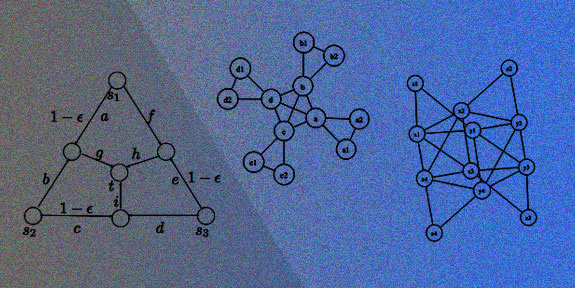# Research > Algorithms & Complexity Theory## Overview

Theoretical Computer Science is concerned with rigorously quantifying the computational constraints on processes. Commonly, theoreticians analyze the runtime, space requirements or communication requirements of algorithms for given computational problems; devise new algorithms; or show lower bounds on the inherent resource requirements of problems. Beyond these types of problems, however, theoreticians also study the limits of computation by quantum devices, evolutionary processes, economic markets, and more.

The theory group at CMS takes the widest possible view of computation. Not only do we study the complexity of fundamental problems from linear algebra, such as matrix multiplication, or graph theory, such as routing problems. But we also take at heart to expand our horizon much beyond the study of classical models. Building on close collaborations with Caltech’s research groups in quantum information, chemistry or biology we pursue the computational theory of physical systems: what kind of computations can a quantum system, a chemical molecule, a biological cell, or an abstract social network, perform? What does answering this question tell us about the underlying system?

## Faculty

Related research groups & Centers > CMI, CS Theory group, DOLCIT, IQIM, MPP, Paradise, RSRG, SISL

## Related Courses

CS 21. Decidability and Tractability.
CS 38. Introduction to Algorithms.
ACM/CMS 104. Linear Algebra and Applied Operator Theory.
ACM/CMS 113. Introduction to Optimization.
ACM/CS 114. Parallel Algorithms for Scientific Applications.
ACM/EE/CMS 116. Introduction to Stochastic Processes and Modeling.
CS 116. Reasoning about Program Correctness.
Ma/CS 117 abc. Computability Theory.
CS 118. Logic Model Checking for Formal Software Verification
EE/Ma/CS 126 ab. Information Theory.
EE/Ma/CS 127. Error-Correcting Codes.
CS/EE/Ma 129 abc. Information and Complexity.
Ec 135. Economics of Uncertainty and Information.
CS 138 abc. Computer Algorithms.
CS/EE/CMS 144. Networks: Structure & Economics.
CS/EE 147. Network Performance Analysis.
SS/CS 149. Introduction to Algorithmic Economics
CS 150. Probability and Algorithms.
CS 151. Complexity Theory.
CS/SS 152. Introduction to Cryptography.
CS 153. Current Topics in Theoretical Computer Science
CS/CNS/EE 154. Artificial Intelligence.
CS/CNS/EE 155. Machine Learning & Data Mining
CS/CNS/EE 156 ab. Learning Systems.
ACM/EE/CMS 170. Mathematics of Signal Processing.
PS/Ec 172. Game Theory.
Ec 181. Convex Analysis and Economic Theory.
BE/CS/CNS/Bi 191 ab. Biomolecular Computation.
CNS/Bi/Ph/CS 187. Neural Computation.
CNS/CS/EE 188. Topics in Computation and Biological Systems.
ACM/CS/EE/CMS 218. Statistical Inference.
Ph/CS 219 abc. Quantum Computation.